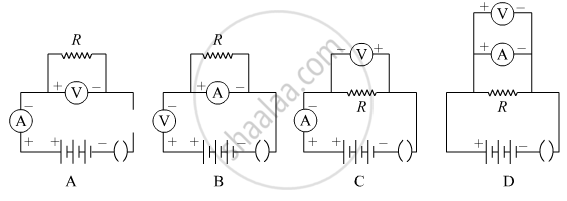# Which one of the following is the correct set-up for studying the dependence of the current on the potential difference across a resistor and why? - Science

Short Note

Which one of the following is the correct set-up for studying the dependence of the current on the potential difference across a resistor and why?#### Solution

In this experiment of determining the dependence of the current on the potential difference across a resistor the voltmeter should be connected in parallel to the resistor and the ammeter should be connected in series with the resistor. So, in the given set-ups, A is the correct one because the voltmeter is in parallel to the resistor and the ammeter is in series with the resistor, and secondly, the polarities of ammeter and voltmeter are also correct.
Concept: Electric Potential (Electrostatic Potential) and Potential Difference
Is there an error in this question or solution?